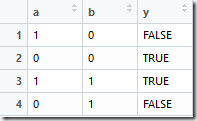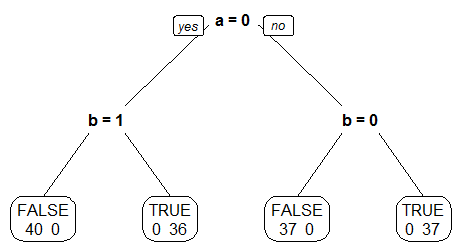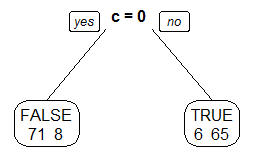## Sunday, April 24, 2016

### Why doesn’t my decision tree recognize a simple pattern?

Data Mining algorithms are sometimes perceived to be extremly intelligent and established with many super powers, humans don’t have. In today’s post I’d like to show a simple example in which a mining model fails to detect a very simple rule in the data. This illustrates the need for feature engineering not only to enhance the data with new associations but also to include simple calculations, for example deltas instead of absolute values.

I’m starting with a very simple pattern of two variables (a, b) and a single output (y):As you can see, “Y” equals TRUE, if “A” and “B” are identical (boolean operation is “not xor”). So, this is a very simple pattern, which should be easily detected by a data mining algorithm. In order to use this data as source for an algorithm, I manifolded this pattern 50 times to result in 200 rows of data with this pattern. In R this reads as follows:

> newdata <- data.frame(
"a"=as.factor(c(1,0,1,0)),
"b"=as.factor(c(0,0,1,1))
)
> data <- do.call("rbind", replicate(50, newdata, simplify = FALSE))
> data\$y <- as.factor(data\$a==data\$b)

> fit <- rpart(y~.,data)

The result shows that no split has been detected in the tree or – in other words – the rpart model didn’t understand our simple rule:

n= 200

node), split, n, loss, yval, (yprob)
* denotes terminal node

1) root 200 100 FALSE (0.5000000 0.5000000) *

Instead, all observations are treated as “FALSE” in our root node. Consequently, if we ask the model to do the prediction, it always responds with the answer “false”:

> cbind(newdata,predict(fit,newdata, type="class"))

a b predict(fit, newdata, type = "class")
1 1 0                                 FALSE
2 0 0                                 FALSE
3 1 1                                 FALSE
4 0 1                                 FALSE

The reason for this behavior is easy to understand if you look at the decision the model has to make for the first split. Since our data cases are perfectly balanced, neither “A” nor “B” seems to have any effect on the output. For example, if “A” equals zero you find “Y” having 50% true and 50% false (see the table from above). The same situation is seens with “A” equals one. And the same situation is true for “B”. So the feature selection algorithm decides that none of our input variables has a univerariate influence on the output variable.

> table(data\$a, data\$y)

FALSE TRUE
0    50   50
1    50   50

So the reason for our decision tree not detecting the pattern is the unnatural perfectly balanced structure of the data. If we use a random forest instead, the draws wouldn’t be equally balanced and the algorithm would detect the pattern:

> fit <- randomForest(y~.,data)
> plot(fit)
> cbind(newdata,predict(fit,newdata, type="class"), predict(fit,newdata, type="prob"))

a b predict(fit, newdata, type = "class") FALSE  TRUE
1 1 0                                 FALSE 0.728 0.272
2 0 0                                  TRUE 0.264 0.736
3 1 1                                  TRUE 0.218 0.782
4 0 1                                 FALSE 0.752 0.248

Ok, this looks much better. And also for our decision tree, if we take a sample (75% of the data rows resulting in 150 rows for this example), the result looks much better. Here’s the resulting tree:As you can see, the leaf nodes are “perfect” with no error. So our pattern has been detected in this case. Of course you wouldn’t expect such a perfect distribution as I constructed for this demonstration, but still this effect could be an issue for your model. As it is unlikely to have such a balanced distribution in real life, it is as likely that you’re dealing with more than just two input variables. So, let’s add a third variable which approximates “Y” with a success rate of 90%:

> data\$c<-as.factor(ifelse(data\$y==TRUE,ifelse(runif(n=nrow(data))<=.9,1,0),ifelse(runif(n=nrow(data))<=.9,0,1)))

Now “C” is a good but not perfect approximation of “Y”. Let’s retrain the tree using “A”, “B” and “C” as input variables. As you can see from the tree visualization below, the algorithm chose the “easy” way and used our approximation as the only split condition, instead of detecting the pattern (as in the case where “C” was missing):As a consequence our true prediction rate goes down from 100% (pattern detected) to about 90% (approximation variable used). 14 out of 150 cases failed. Ok, this is still good but the point is, that we’re getting better results if we think about the way splits are calculated and therefore add additional attributes (in this case we could have added “A==B” as a helper attribute) to support the decisions. There are many more examples:

• Instead of absolute values (temperature 26°, 28°, 32°) it might be better to add relative (delta) values (temperature delta 2°, 4°)
• Instead of two absolute variables (energy consumption and workload) it might be a good idea to also add the ratio (energy consumption / workload)
• Difference/Change of a value compared to the average (or a regression) of a given time range before (for example, relative change of a value compared to the linear regression of the last 3 hours).
• As shown in the example above: for two variables it might be good to add a measure of similarity (equal or almost equal)

Conclusion

Data Mining algorithms like the decision tree sometimes struggle with simple patterns in data. Instead of feeding the data “as it is” into the algorithm, it’s often a good idea to add some derived information (delta, ratio, correlation, measure of equality etc.) to support the model.